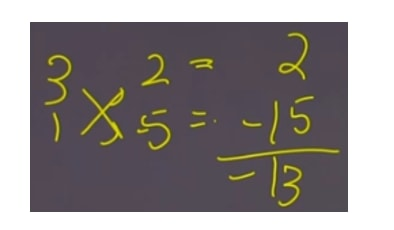Solving polynomial equations - Operations with Polynomials

What is the zero product property

The zero product property definition tells us that if:

a x b = 0

then either a or b has to equal 0.

Logically, this makes sense. Any number multiplied by 0 gives us 0. No other combinations can give us this. Therefore, one of the two unknown numbers must be 0.

How to solve polynomials

When we're asked to solve a polynomial, we're actually being asked to find the roots where the function equals 0. You can see how the above zero product property can come in handy! So how do we start solving polynomials? We'll have to do some isolating.

How to isolate a variable

In order to figure out when a polynomial equals 0, we'll have to isolate the variable that is in the equation so that we can find its value. This is like how we solve two-step linear equations. Let's try it out with a simple polynomial equation. Do you know how to solve for x?

$2x - 1 = 0$

Since we don't know what x is, we'll have to isolate it onto one side to see what it equals. In this case, let's start with moving the 1, and then the 2 that's next to the x.

$2x - 1 = 0$

$2x = 1$

$x = \frac{1}{2}$

When you've got a positive on one side and you've got to move it to the other side of the equal sign, simply reverse the sign to negative. If it's negative and you've got to move it, it'll become positive. Similarly, if you have to multiply on one side and now you're trying to move it to the other side, you'll have to divide it. If you've got a division, it'll become multiplication on the other side of the equal sign.

If you've got a variable on both sides of the equal sign, you'll still have to group it to one side in order to find its value.

Example problems

Question 1:

1a) Solve the equation:

$4x^{2} - 9 = 0$

Solution:

Since we're dealing with a degree of a polynomial (in this case it's the power of 2), when you move a square to the other side of the equal sign, you'll have a square root. A note is that all positive real numbers has two square roots—one that's positive and one that's negative. Therefore, we'll put a ±sign in front of the square root to indicate we'd like both the negative and positive in our final answer.

$4x^{2} = 9$

$x^{2} = \frac{9}{4}$

$x = \pm \sqrt{\frac{9}{4}}$

$x = \pm \frac{3}{2}$

1b) Solve the equation:

$(2x - 3)^{2} = 1$

Solution:

$2x - 3 = \pm \sqrt{1}$

$2x - 3 = \pm 1$

$2x = \pm 1 + 3$

$x = \frac{3 \pm 1}{2}$

$x = 2, 1$

Question 2

Solving the equation:

$3x^{2} - 13x - 10 = 0$

We have to factor the polynomial before solving for x so that we can get it into a form that looks like we're about to do distributive property on it.Factorize the equation to get the values of x

After factoring, we have:

$(3x + 2)(x - 5) = 0$

To solve for x, we can separate $(3x + 2)(x - 5) = 0$ into two equations using the zero product property:

$(3x + 2) = 0$,and $(x - 5) = 0$

Isolate and solve for x

$3x + 2 = 0$

$3x = -2$

$x = \frac{-2}{3}$

$x - 5 = 0$

$x = 5$

So, in this question, $x = \frac{-2}{3}$ and $x = 5$

Not sure about your answers? Here's an online polynomial equation calculator you can use to check your answer when solving polynomial equations.

Want to move on? You can take a look at these related lessons to improve on your understanding:

Solving polynomial equations

Depending on the degree what terms are included in the polynomial equations, you may simply move terms around to get the answers. Sometimes, you may need to perform factoring in order to solve the equations. Yet, the rule of thumb is always isolating the unknown to one side of the equation.

Lessons

Solving the equations: